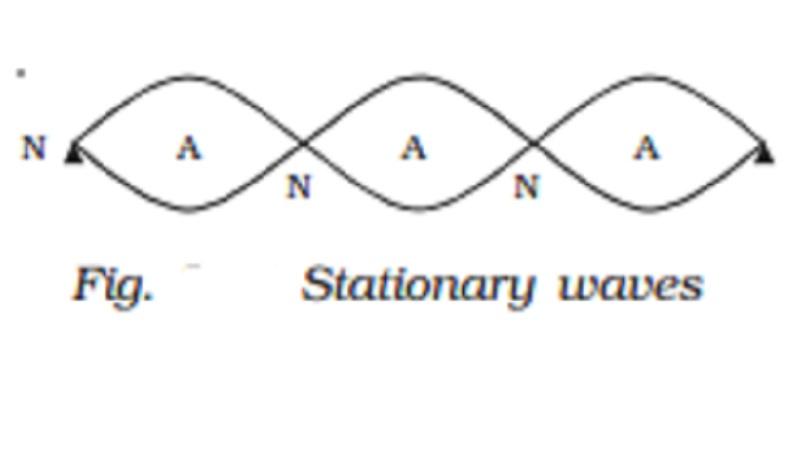Home | | Physics | | Physics | Analytical method and Characteristics of stationary waves

# Analytical method and Characteristics of stationary wavesWhen two progressive waves of same amplitude and wavelength travelling along a straight line in opposite directions superimpose on each other, stationary waves are formed.

Stationary waves

When two progressive waves of same amplitude and wavelength travelling along a straight line in opposite directions superimpose on each other, stationary waves are formed.

Analytical method

Let us consider a progressive wave of amplitude a and wavelength travelling in the direction of X axis.

y1 = a sin 2π( t/T -  x/ λ )            ????(1)

This wave is reflected from a free end and it travels in the negative direction of X axis, then

y2 = a sin 2π( t/T -  x/ λ )            ????(2)

According to principle of superposition, the resultant displacement is

y = y1 + y2

=a[sin 2π( t/T -  x/ λ ) + sin 2π( t/T +  x/ λ ) ]

=a[2 sin(2πt/T) cos(2πx/ λ)]

y = 2a cos(2πx/ λ) sin(2πt/T)   ?????.(3)

This is the equation of a stationary wave.

(i) At points where x = 0, λ/2 , λ, 3λ/2. the values of cos(2πx/ λ)= ?1

A = + 2a. At these points the resultant amplitude is maximum. They are called antinodes (Fig. 7.13).

(ii) At points where x = λ/4, 3 λ/4, 5 λ/4 ?????? the values of cos (2πx / λ) = 0

A = 0. The resultant amplitude is zero at these points. They are called nodes (Fig.).

The distance between any two successive antinodes or nodes is equal to λ/2  and the distance between an antinode and a node is  λ/4.

(iii) When t = 0, 2, T/2,T,3T/2,2T,???then sin(2πx / T) = 0 the displacement is zero.

(iv) When t = T/4 , 3T/4, 5T/4, etc??.. sin(2πt/T) = ? 1, the displacement is maximum.

Characteristics of stationary waves

1.  The waveform remains stationary.

2.  Nodes and antinodes are formed alternately.

3. The points where displacement is zero are called nodes and the points where the displacement is maximum are called antinodes.

4.   Pressure changes are maximum at nodes and minimum at antinodes.

5.   All the particles except those at the nodes, execute simple harmonic motions of same period.

6.  Amplitude of each particle is not the same, it is maximum at antinodes decreases gradually and is zero at the nodes.

7.  The velocity of the particles at the nodes is zero. It increases gradually and is maximum at the antinodes.

8.  Distance between any two consecutive nodes or antinodes is

equal to λ2 , whereas the distance between a node and its adjacent antinode is equal to λ4 .

9.  There is no transfer of energy. All the particles of the medium pass through their mean position simultaneously twice during each vibration.

Particles in the same segment vibrate in the same phase and between the neighbouring segments, the particles vibrate in opposite phase.

Study Material, Lecturing Notes, Assignment, Reference, Wiki description explanation, brief detail

Related Topics